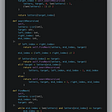# Generate Random Point in a Circle — Day 98(Python)

Today’s question is a Math based question. It is taken from the Daily leetcode coding challenge- March edition. Let us look into the problem statement.

478. Generate Random Point in a Circle

Given the radius and x-y positions of the center of a circle, write a function `randPoint` which generates a uniform random point in the circle.

Note:

1. input and output values are in floating-point.
2. radius and x-y position of the center of the circle is passed into the class constructor.
3. a point on the circumference of the circle is considered to be in the circle.
4. `randPoint` returns a size 2 array containing x-position and y-position of the random point, in that order.

Example 1:

`Input: ["Solution","randPoint","randPoint","randPoint"][[1,0,0],[],[],[]]Output: [null,[-0.72939,-0.65505],[-0.78502,-0.28626],[-0.83119,-0.19803]]`

Example 2:

`Input: ["Solution","randPoint","randPoint","randPoint"][[10,5,-7.5],[],[],[]]Output: [null,[11.52438,-8.33273],[2.46992,-16.21705],[11.13430,-12.42337]]`

Explanation of Input Syntax:

The input is two lists: the subroutines called and their arguments. `Solution`'s constructor has three arguments, the radius, x-position of the center, and y-position of the center of the circle. `randPoint` has no arguments. Arguments are always wrapped with a list, even if there aren't any.

As an input, we have 2 co-ordinates i.e X and Y. These 2 input values are the coordinates for the center. We have another input which is the radius of the circle. We need to generate random points which fall within the circle.

Since we need to generate random uniform numbers, we will be using random.uniform function in Python. We require 2 parameters i.e min value and max value, that will act as input to random.uniform(). How can we find the min and max values for a circle?

Since we have a center coordinate and radius given, we will leverage this value to find the min and max values.

`self.x_min = x_center - radiusself.x_max = x_center + radiusself.y_min = y_center - radiusself.y_max = y_center + radius`

Once we find the min and max values, we will use random.uniform() to generate uniform coordinates.

One check to be done is to check if the values fall within the circle. We have a mathematical formula that checks if a point falls within the circle.

Let us look into the code snippet.

`import randomclass Solution:   def __init__(self, radius: float, x_center: float, y_center: float):        self.x_center = x_center        self.y_center = y_center        self.x_min = x_center - radius        self.x_max = x_center + radius        self.y_min = y_center - radius        self.y_max = y_center + radius        self.radius = radius   def randPoint(self) -> List[float]:        output = [0, 0]        while True:            output, output = random.uniform(self.x_min, self.x_max), random.uniform(self.y_min, self.y_max)            if sqrt(pow(output-self.x_center,2) + pow(output-self.y_center,2)) <= self.radius:                return output# Your Solution object will be instantiated and called as such:# obj = Solution(radius, x_center, y_center)# param_1 = obj.randPoint()`

# Complexity analysis.

Time Complexity

On average the time complexity is O(1) since we are expecting random to generate values within the circle. In the worst case, this can go up to infinity too.

Space Complexity

The space complexity is O(1) since we are not using any other data structure to store calculations.

--

--

--

## More from Annamariya Tharayil

Software Engineer. Find me @ www.linkedin.com/in/annamariya-jt

Love podcasts or audiobooks? Learn on the go with our new app.

## timeghost and Zapier## Building UI in Unity## Consistency > intensity## Serving files in current directory using Python http module## Embed<IOTA> update III## Machine Learning Book Bundle## Annamariya Tharayil

Software Engineer. Find me @ www.linkedin.com/in/annamariya-jt

## CaDS— Code and Data Society## LeetCode Patterns Adventure 18 — Find Smallest Letter Greater Than Target